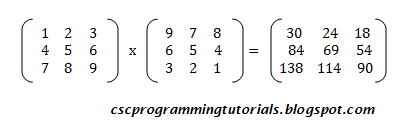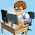# 3x3 Matrix Multiplication Using Pascal ProgrammingThe program below computes the product of two 3 by 3 matrices.

```PROGRAM matrix (Input, Output);
VAR
A, B, C: ARRAY[1..3, 1..3] OF Integer;
Arow, AColumn, Brow, BColumn, I, J, K, SUM: Integer;
BEGIN
Write(' ':4O, 'MATRIX MULTIPLICATION PROGRAM');
Write(' ':4O, '-----------------------------');
Writeln;}
Writeln(' Enter the number of ROWS of Matrix A');
Writeln(' Enter the number of COLUMNS of Matrix A');
Writeln(' Enter the number of ROWS of Matrix B');
Writeln(' Enter the number of COLUMNS of Matrix B');
Writeln();
IF (AColumn = Brow) THEN
BEGIN
Writeln(' Enter the elements of Matrix A');
For I:= 1 to 3 DO
For J:= 1 TO 3 DO
Writeln(' Enter the elements of Matrix B');
For I:= 1 to 3 DO
For J:= 1 TO 3 DO<
Writeln;
{ Display the elements of Matrix A }
Writeln(' MATRIX A');
Writeln(' --------');
For I:= 1 TO 3 DO
BEGIN
For J:= 1 TO 3 DO
Write(A[I,J]:3);
Writeln;
END;
Writeln;
{ Display the elements of Matrix B }
Writeln(' MATRIX B');
Writeln(' --------');
For I:= 1 TO 3 DO
BEGIN
For J:= 1 TO 3 DO
Write(B[I,J]:3);
Writeln;
END;
Writeln;
{ Compute the Product of Matrices A and B }
For I:= 1 to 3 DO
For J:= 1 TO 3 DO
BEGIN
SUM:=0;
For K:= 1 TO 3 DO
SUM:= SUM + A[I,K] * B[K,J];
C[I,J]:= SUM;
END;
Writeln;
{ Display the Product of Matrices A and B }
Writeln(' MATRIX AB');
Writeln(' ---------');
Writeln;
For I:= 1 TO 3 DO
BEGIN
For J:= 1 TO 3 DO
Write(C[I,J]:5);
Writeln;
END;
END
ELSE
Writeln(' Matrix A and B cannot be multiplied. Enter Matrices in the order m x n and n x p');
Writeln();
Writeln(' PRESS THE ENTER KEY TO EXIT');
END.
```Sample Program Run
You can modify the program if you like. Some ideas are:
1. Modify the program using Functions/Procedures.
2. Modify the program such that it can compute the product for any size of two matrices provided the number of columns of first matrix is equal to the number of rows of the second matrix.

1.When I do the multiplication, I get
[30 23 19 ]
[84 65 58 ]
[138 107 97 ]
What could be going on that my calculation is close but differs from yours?

1.This site agrees with me:

2.Hi Tom. I just rechecked the program and got the same answer as before. You can view the screenshot here - http://goo.gl/d06oNH I guess the problem is your input. Check! In this case, when prompted to enter the elements for matrix A, type 1 then press ENTER, type 2 and press ENTER, to 9. For matrix B, type 9 then press ENTER, type 8 and press ENTER, to 1. I also solved the problem manually on paper just to make sure it was correct. Also check your code.

3.Yr calculation is right.. no error but the above calculation answer's given wrong

2.I found the difference: I was going by your image, which has 978 instead of 987 in the top row. Funny :D

3.As Tom says, the image is incorrect and lists the first row as 978, but the result is calculated from a first row of 987. The sample run shows the correct input.

DISCLAIMER: Opinions expressed in comments are those of the comment writers alone and does not reflect or represent the views of the post author. We reserve the right to delete any post deemed inappropriate or offensive and/or spammy. Please do not use abusive words/hate speech.

I Appreciate your valuable Feedback. So, Please DO NOT SPAM - Spam comments will be deleted immediately.

Don't use brand name in name field and you're not allowed to use links in comments unless it's necessary. Such comments will be removed immediately. To get notified of replies or follow-up comments, click the box next to notify me.

Thanks.